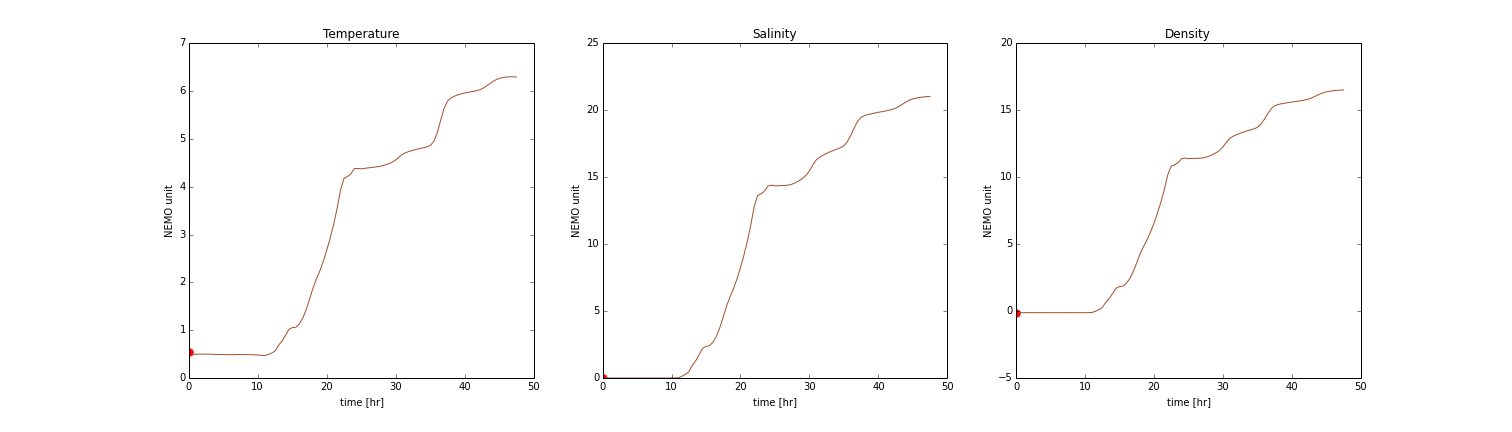# Tracers with Ariane¶

In addition to a particle’s trajectory (longitude and latitude) and depth, Ariane can also help us analyze tracers along the particle’s trajectory.

• Temperature

• Salinity

• Density

We will be making changes in namelist.

Ariane requires both salinity and temperature data as input. It also requires density data or an indication that density should instead be calculated using salinity and temperature.

Our model produces files with filenames that follow this format: SalishSea_t_yyyymmdd_yyyymmdd_grid_T.nc. These files contain salinity and temperature. Therefore, Ariane will be calculating density.

Add the following sections to namelist. Remember to modify the directory and filename of your input files.

### Temperature¶

```&TEMPERAT
c_dir_te ='/ocean/nsoontie/MEOPAR/SalishSea/results/storm-surges/final/dec2006/all_forcing/30min/',
c_prefix_te ='SalishSea_30m_20061214_20061215_grid_T.nc',
ind0_te =-1,
indn_te =-1,
maxsize_te =-1,
c_suffix_te ='NONE',
nc_var_te ='votemper',
/
```

### Salinity¶

```&SALINITY
c_dir_sa ='/ocean/nsoontie/MEOPAR/SalishSea/results/storm-surges/final/dec2006/all_forcing/30min/',
c_prefix_sa ='SalishSea_30m_20061214_20061215_grid_T.nc',
ind0_sa =-1,
indn_sa =-1,
maxsize_sa =-1,
c_suffix_sa ='NONE',
nc_var_sa ='vosaline',
/
```

votemper and vosaline are the variable names for temperature and salinity in our input file.

Add the following parameters to the ARIANE section of the namelist:

• key_computesigma: False if density is read into Ariane; True if Ariane calculates density.

• zsigma: Reference depth for calculation of density.

And change:

• key_alltracers: True if tracers are required.

### Ariane¶

```&ARIANE
key_alltracers =.TRUE.,
key_sequential =.FALSE.,
key_ascii_outputs =.TRUE.,
mode ='qualitative',
forback ='forward',
bin ='nobin',
init_final ='init',
nmax =5,
tunit =3600.,
ntfic =1,
tcyc =0.,
key_computesigma =.TRUE.,
zsigma =1.,
/
```

## Results¶

The variables names for the tracers are:

• traj_temp

• traj_salt

• traj_dens

### Plots¶

The results produced for the example above: